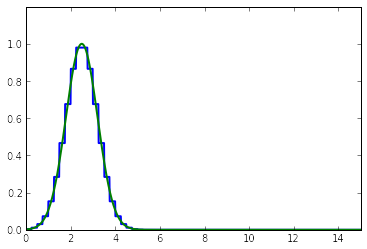# Advection Equation and the REA algorithm¶

by Mauricio J. Del Razo S. 2014

In this notebook we will develop and explain the REA (reconstruct, evolve and average algorithm) for the advection equation. Since any homogeneous hyperbolic linear system of equations can be decoupled into a system of advection equations, this finite volume method algorithm is a fundamental block in the numerical solution of hyperbolic problems. The ideas presented with this algorithm will remain to be relevant for non-linear systems.

## 1. Decoupling into a system of advection equations¶

Consider a system of one dimensional linear hyperbolic equations, written in its first order form,

$$\bar{q}_t + \mathbf{A} \bar{q}_x = 0.$$

We can decompose the matrix $\mathbf{A} = \mathbf{R \Lambda R^{-1}}$, as the product of the matrix of eigenvectors $\mathbf{R}$ with the diagonal matrix of eigenvalues $\mathbf{\Lambda}$ and the inverse of $\mathbf{R}$. Substituiting into the previus equation and multipling by $\mathbf{R^{-1}}$ on the left side, we obtain

$$\mathbf{R^{-1}} \bar{q}_t + \mathbf{\Lambda R^{-1}} \bar{q}_x = 0.$$

We can do the change of variable $\bar{w} = \mathbf{R^{-1}}\bar{q}$, and as $\mathbf{R^{-1}}$ is constant in time and space, we obtain,

$$\bar{w}_t + \mathbf{\Lambda} \bar{w}_x = 0,$$

where $\mathbf{\Lambda}$ is the diagonal matrix of eigenvalues. This yields a system of uncoupled advection equations, where the propagation speeds correspond to the eigenvalues of $\mathbf{\Lambda}$ or $\mathbf{A}$. This means that if we can solve an advection equation numerically, we can solve the original system of hyperbolic equations.

## 2. The REA algorithm for the advection equation¶

Consider now a simple one dimensional advection equation for some quantity $q$,

$$q_t + c q_x = 0, \\[3mm] q(x,0) = q_0(x).$$

It has solution $q(x,t) = q_0(x-ct)$, like shown below,We would like to develop a numerical method that solves this equation and conserves the quantity $q$. We will first need to divide the space into grid cells $\mathcal{C_i}=(x_{i-1/2},x_{i+1/2})$ separated by $\Delta x$ and consider the cell averages of $q$ to be,

$$Q_i^n = \frac{1}{\Delta x} \int_{x_{i-1/2}}^{x_{i+1/2}} q(x,t_n) dx$$

Once this is decided, we can begin the REA algorithm (for a detailed explanation see LeVeque 2002 ):

1. Reconstruct a piecewise polynomial function $\bar{q}^n(x,t_n)$ from the cell averages $Q_i^n$. The simplest possibility is a piecewsie constant $\bar{q}^n(x,t_n) = Q_i^n$. More complicated approximations could be useful for better accuracy.2. Evolve the advection equation a time $\Delta t$ with the reconstruction as initial data to obtain $\bar{q}^{n+1}(x,t_{n+1})$3. Average $\bar{q}^{n+1}(x,t_{n+1})$ over the cells to obtain new cell averages, $$Q_i^{n+1} = \frac{1}{\Delta x} \int_{x_{i-1/2}}^{x_{i+1/2}} \bar{q}^{n+1}(x,t_{n+1}) dx$$## 3. A python implementation¶

In this section, we will implement the REA algorithm for the advection equation. The code is well commented, so it can be easily followed by the reader.

In :
# Import some libraries
import numpy as np

# Set advection speed (c in PDE)
c = 10.0

# Set spatial domain
xmin = 0
xmax = 15

# Define q0(x), initial function to be advected
def q0(x):
qq = np.exp(-(x-2.5)*(x-2.5))
return qq

# Create a function for the REA algorithm (parameter: number of cells and final time)
def REA(ncells,tfinal):

# Create grid edges, space step and init time
x = np.linspace(xmin,xmax,ncells+1) # grid edges
dx = x - x
time = 0

# Define time step size obeying CFL condition
dt = dx/(2.0*c)

# Initialize solution q(x,t): q
q = np.zeros(ncells)

# Calculate cell averages at time 0 (using q0 function)
Qavg = np.zeros(ncells)
for i in range(ncells):
# Integrate q0 function in the grid intervals
Qavg[i] = integral/dx

# Initialiaze REA algorithm
while (time <= tfinal):
# RECONSTRUCT STEP
q = 1.0*Qavg

# EVOLVE and AVERAGE STEP (move by dt and average)
time = time + dt
for i in range(ncells):
if (c>=0):
Qavg[i] = (c*dt*q[i-1] + (dx-c*dt)*q[i])/dx
else:
Qavg[i] = (c*dt*q[i+1] + (dx-c*dt)*q[i])/dx

# If final time close adjust dt to match desired final time
if (time > tfinal):
dt = tfinal - (time - dt)
for i in range(ncells):
Qavg[i] = (c*dt*q[i-1] + (dx-c*dt)*q[i])/dx
q = Qavg

return (x,q)

# Function to calculate exact solution for comparison
def q_exact(tfinal):
# Calculate dense x
xx = np.linspace(xmin,xmax,1000)
# Obtain exact solution
qexact = q0(xx-c*tfinal)
return (xx,qexact)

In :
#Import plotting libraries
%matplotlib inline
import matplotlib.pyplot as plt
from pylab import *
from IPython.html.widgets import interact
from ipywidgets import StaticInteract, RangeWidget

# Create plotting function for REA and exact solution
def plot_REA(ncells, tfinal):
# Create Figure to plot
fig = plt.figure()

# Call REA algorithm and exact solution
x, qREA = REA(ncells,tfinal)
xx, qexact = q_exact(tfinal)

# Transform REA into piecewise function for pretty plotting
qREA_pp = 0*xx
for i in range(len(x)-1):
for j in range(len(xx)):
if (xx[j] >= x[i] and xx[j] < x[i+1]):
qREA_pp[j] = qREA[i]

# Plot solutions
ax.plot(xx,qREA_pp,'-b',linewidth=2)
ax.plot(xx,qexact,'-g',linewidth=2)
ax.axis([min(xx),max(xx),0,1.2])
return fig

# Create animated solution
StaticInteract(plot_REA, ncells=RangeWidget(60,760,100), tfinal=RangeWidget(0,0.8,0.05))

Out: